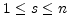Output
Subsections

## Output

If one simulates a network then one also wants to record the quantities of interest. In our introductory example we were interested in the membrane potential of the leaky-integrate and fire neuron. CSIM can record any field of any object by means of Recorder objects. The following code fragment shows how to set up a Recorder to record the membrane potential (Vm) of a LifNeuron object with handle n.


» rec = csim('create','Recorder');
» csim('connect',rec,n,'Vm');

Note that one Recorder object can record an arbitrary number of fields from arbitrary objects.

### Getting results of the last simulation

After a network simulation via a command like


» csim('simulate',Tsim,InputSignal);

the recorded data of the the last simulation Recorder rec can be obtained by the command

» R=csim('get',rec,'traces');

The exact structure of R for the recorder rec depends on the value of the field commonChannels of the Recorder object.

#### commonChannels = 0

In this case (default) R is a struct array with the only field channel which is in turn a struct array with a similar structure as an input signal. That is

• R.channel(j).idx : handle of the object from which field the data was recorded

• R.channel(j).spiking : binary flag (0/1) which determines if data should be interpreted as spike times or as an analog signal

• R.channel(j).dt : time discretization; for analog signals only

• R.channel(j).data : signal data : vector of the analog values or spike times. Note that the data always starts at time t=0.

• R.channel(j).fieldName : name of the recorded field

#### commonChannels = 1

In this case R has two fields:
• R.data : A double array where R.data(j,s) is the s-th recorded value of the j-th field recorded. Note that the data always starts at time t=0. Remark: The number of values which are recorded depends on the field dt of the Recorder object.

• R.info : A struct array where R.info(j).idx is the handle of the object from which the field R.info(j).fieldName is recorded.

### Getting the results of a multi-stimulus simulation

Reminder: The command


» R=csim('get',rec,'traces');

returns only the results of the last simulation. In the case of a multi-stimulus simulation with stimulus array S of length n this command returns only the result of the simulation with stimulus S(n).

To get the results of all n simulations one has to use


» R=csim('simulate',Tsim,S);


In that case R contains the results of all n stimulations. R{r}(s).channel contains the recorded data of the r-th recorder during the s-th simulation (). R{r}(s).channel is of the format as described in Section 3.2.1.